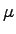Next: Natural Language Processing Up: Lent Term 2009: Part Previous: Computer Vision   Contents

## Digital Signal Processing

Lecturer: Dr M.G. Kuhn

No. of lectures: 12

Prerequisite courses: Probability, Mathematical Methods for Computer Science
The last lecture of Unix Tools (MATLAB introduction) is a prerequisite for the practical exercises. Some of the material covered in Information Theory and Coding and Floating-Point Computation will also help in this course.

Aims

This course teaches the basic signal-processing principles necessary to understand many modern high-tech systems, with a particular view on audio-visual data compression techniques. Students will gain practical experience from numerical experiments in MATLAB-based programming assignments.

Lectures

• Signals and systems. Discrete sequences and systems, their types and properties. Linear time-invariant systems, convolution.

• Phasors. Eigen functions of linear time-invariant systems. Review of complex arithmetic. Some examples from electronics, optics and acoustics.

• Fourier transform. Phasors as orthogonal base functions. Forms of the Fourier transform. Convolution theorem, Dirac's delta function, impulse combs in the time and frequency domain.

• Discrete sequences and spectra. Periodic sampling of continuous signals, periodic signals, aliasing, sampling and reconstruction of low-pass and band-pass signals, spectral inversion.

• Discrete Fourier transform. Continuous versus discrete Fourier transform, symmetry, linearity, review of the FFT, real-valued FFT.

• Spectral estimation. Leakage and scalloping phenomena, windowing, zero padding.

• Finite and infinite impulse-response filters. Properties of filters, implementation forms, window-based FIR design, use of frequency-inversion to obtain high-pass filters, use of modulation to obtain band-pass filters, FFT-based convolution, polynomial representation, z-transform, zeros and poles, use of analog IIR design techniques (Butterworth, Chebyshev I/II, elliptic filters).

• Random sequences and noise. Random variables, stationary processes, autocorrelation, crosscorrelation, deterministic crosscorrelation sequences, filtered random sequences, white noise, exponential averaging.

• Correlation coding. Random vectors, dependence versus correlation, covariance, decorrelation, matrix diagonalization, eigen decomposition, Karhunen-Loève transform, principal component analysis. Relation to orthogonal transform coding using fixed basis vectors, such as DCT.

• Lossy versus lossless compression. What information is discarded by human senses and can be eliminated by encoders? Perceptual scales, masking, spatial resolution, colour coordinates, some demonstration experiments.

• Quantization, image and audio coding standards. A/-law coding, delta coding, JPEG photographic still-image compression.

• MPEG standards. Motion compensation, video coding, MP3.

Objectives

By the end of the course students should

• be able to apply basic properties of time-invariant linear systems

• understand sampling, aliasing, convolution, filtering, the pitfalls of spectral estimation

• be able to explain the above in time and frequency domain representations

• be competent to use filter-design software

• be able to visualize and discuss digital filters in the z-domain

• be able to use the FFT for convolution, deconvolution, filtering

• be able to implement, apply and evaluate simple DSP applications in MATLAB

• apply transforms that reduce correlation between several signal sources

• understand and explain limits in human perception that are exploited by lossy compression techniques

• provide a good overview of the principles and characteristics of several widely-used compression techniques and standards for audio-visual signals